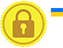# Assignment Operators in javascript

In javascript assignment operator are used to assign a value to a variable.

S.No Symbol Name Description Example
`=` Assignment It is used to assign values to the given variable. Try Yourself
`+=` Addition assignment It is used to add the right side value with the value of left side variable and store the result in the same variable. Try Yourself
`-=` Subtraction assignment It is used to subtract the right side value with the value of left side variable and store the result in the same variable. Try Yourself
`*=` Multiplication assignment It is used to multiply the right side value with the value of left side variable and store the result in the same variable. Try Yourself
`/=` Division assignment It is used to divide the right side value with the value of left side variable and store the quotient in the same variable. Try Yourself
`%/` Remainder assignment It is used to divide the right side value with the value of left side variable and store the remainder in the same variable. Try Yourself
`<<=` Left shift assignment
• In first step, we assign a value to a variable which then internally converts it into binary value(eg- 25 => 11001).
• In second step, a value is given(3) which denotes the number of positions the binary value is to be shifted left side and zero is added at those positions (11001000).
• Shifted binary value is converted into numerical value(200).
Try Yourself
`>=` Right shift assignment
• In first step, we assign a value to a variable which then internally converts it into binary value(eg- 25 => 11001).
• In second step, a value is given(3) which denotes the number of positions the binary value is to be shifted right side(11) by removing the values at the right end.
• Shifted binary value is converted into numerical value(3).
Try Yourself
`>>>=` Unsigned right shift assignment
• In first step, we assign a value to a variable which then internally converts it into binary value(eg- -25 => -11001).
• In second step, a value is given(3), it returns(11111111111111111111111111100).
• Shifted binary value is converted into numerical value(536870908).
• The sign bit will be converted to zero inorder to set the value non negative.
Try Yourself
`&=` Bitwise AND assignment It converts the given numerical values into binary value, and does binary AND opertaion, and the result will be stored in the left side variable. Try Yourself
`^=` Bitwise XOR assignment It converts the given numerical values into binary value, and does binary XOR opertaion, and the result will be stored in the left side variable. Try Yourself
`|=` Bitwise OR assignment It converts the given numerical values into binary value, and does binary OR opertaion, and the result will be stored in the left side variable. Try Yourself